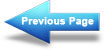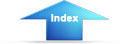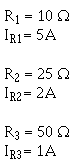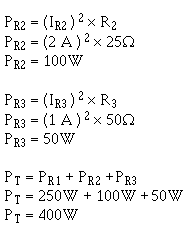Custom SearchResistance in a Parallel CircuitMatter, Energy, and ElectricityEquivalent CircuitsPower in a Parallel Circuit
Power computations in a parallel circuit are essentially the same as those used for the series circuit. Since power dissipation in resistors consists of a heat loss, power dissipations are additive regardless of how the resistors are connected in the circuit. The total power is equal to the sum of the power dissipated by the individual resistors. Like the series circuit, the total power consumed by the parallel circuit is:Example. Find the total power consumed by the circuit in figure 3-48.

Figure 3-48. - Example parallel circuit.Given:Solution:Since the total current and source voltage are known, the total power can also be computed by:

Given:Solution:Integrated Publishing, Inc. - A (SDVOSB) Service Disabled Veteran Owned Small Business